• Introduction
• What is in this manual
• What is Caspoc
• User interface
• Introduction
• Starting
• Simulation
• Editing
• Viewing and printing
• Getting Started
• Basic editing
• Simulation in the time domain
• Basic User Interface Topics
• Editing
• Simulation
• Viewing
• Library
• Reports
• Project management
• Circuit and Block Diagram Components
• Introduction
• Cscript and user defined functions
• Component parameters
• Modeling Topics
• Introduction
• Power Electronics
• Semiconductors
• Electrical Machines
• Electrical drives
• Power Systems
• Mechanical Systems
• Thermal Systems
• Magnetic Circuits
• Green Energy
• Coupling to FEM
• Experimenter
• Analog hardware description language
• Embedded C code Export
• Coupling to Spice
• Small Signal Analysis
• Matlab coupling
• Tips and tricks
• Appendices

## Using the block Signal.

The block SIGNAL is used to create wave forms like square waves, sinusoidal and triangular waveforms. Select the block TIME and SIGNAL from Components/Blocks/Source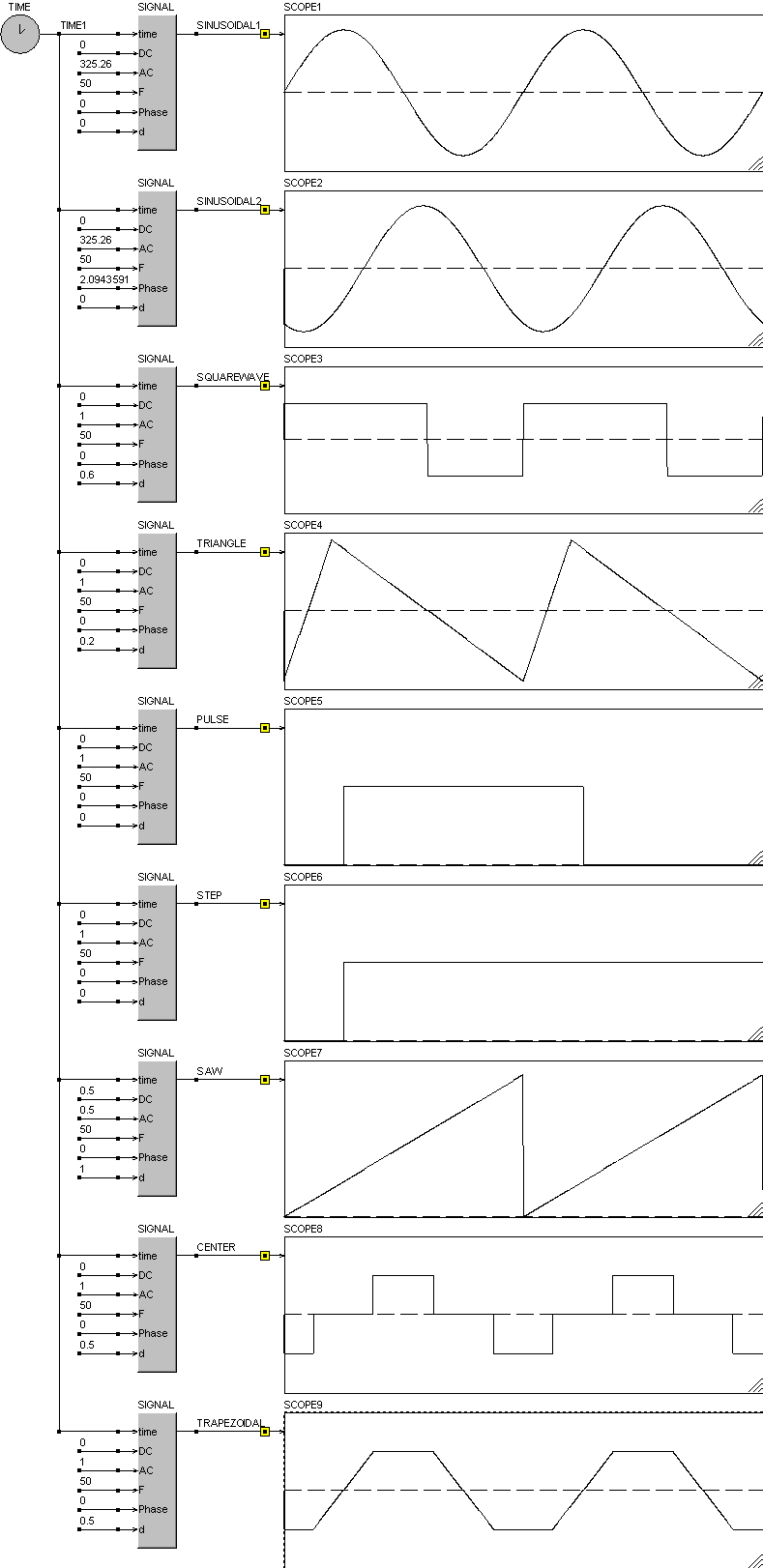The block SIGNAL is used for creating the sinusoidal wave form. Open the block SIGNAL by clicking it with the right mouse button. The third parameter indicates the type of the signal. There are various wave forms possible such as sinusoidal, square wave, triangular, etc. Change the third parameter into 3 to select a sinusoidal wave form.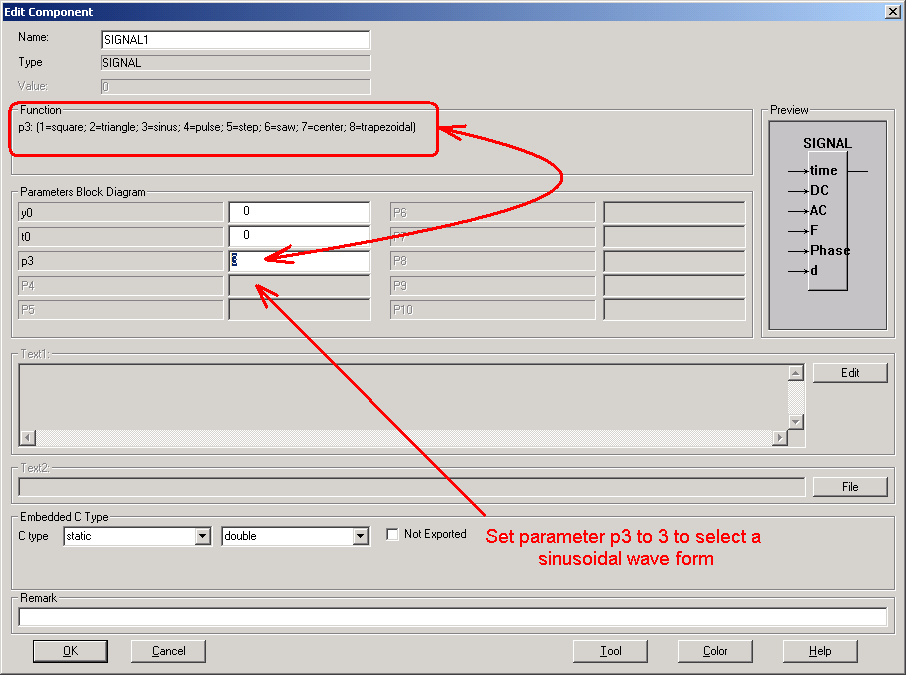Please note that in most cases the first input has to be connected to the block Time. The type of the waveform is defined by parameter p3. Open the component properties by clicking the block Signal with the right mouse button.

The phase in the block Signal is measured in radians (0 .. 2*π) It changes the phase of a sinusoidal signal, but also of the other periodic signals like the square wave and traingular. If the phase is set to zero, the sinusoidal starts from the origin at zero, if it is nonzero, the sinusoidal is sin(2*π*frequency*time-phase) at starts at time zero with sin(-phase).

The following simulation shows various wave forms that can be created using the signal block.The parameters for the block SIGNAL are defined in the reference guide and the online help. Below is an excerpt from the reference guide:

Signal generator
Inputs(6)time DC AC F Phase d
Parameters(3)y0 t0 p3
Defaulty0=0, t0=0, p3=1,
Select fromComponents/Blocks/Source
FunctionInputs:
 Input Type Description 1 T Connect this input to the output of the block Time (s) 2 DC DC Offset 3 AC Amplitude of the signal 4 F Frequency of the signal (Hz) 5 Phase Phase of a repetitive signal (Rad) 6 d Duty Cycle of a repetitive signal (0..1)
Parameters:
 Parameter Type Description p1 Y(0) Initial value of the block signal if t
Waveform:
 Parameter Value Waveform Definition p3 1 Squarewave Repetitive squarewave with frequency F and duty cycle d. The duty cycle d defines how long the amplitude is positive. p3 2 Triangle Repetitive triangle waveform with frequency F and duty cycle d. The duty cycle d defines how long the waveform is rising from DC-AC to DC+AC. Set d=1 to create a rising sawtooth. Set d=0 to create a falling sawtooth p3 3 Sinusoidal y=DC+AC*sin(2*PI*F*t-Phase) p3 4 Pulse For t<1/F the ouput equals DC+AC, if t>1/F the output equals DC p3 5 Step If t>Tdelay, the output becomes DC+AC p3 6 Saw Repetitive triangle waveform with frequency F and duty cycle d. The duty cycle d defines how long the waveform is rising from DC-AC to DC+AC. p3 7 Center Centered square wave. The width of pulse of the squarewave is defined by the input d. For d=1, the output is a squarewave. For d <1 the width of pulse of the squarewave is equal to (1/2)*(1/F)*d. p3 8 Trapezoidal Centered trapezoidal wave. The width of maximum part of the waveform is defined by the input d. For d=1, the output is a squarewave. For d <1 the width of maximum part of the wave is equal to (1/2)*(1/F)*d. For d=0, the output is a triangle waveform.

SCOPE1:P3=3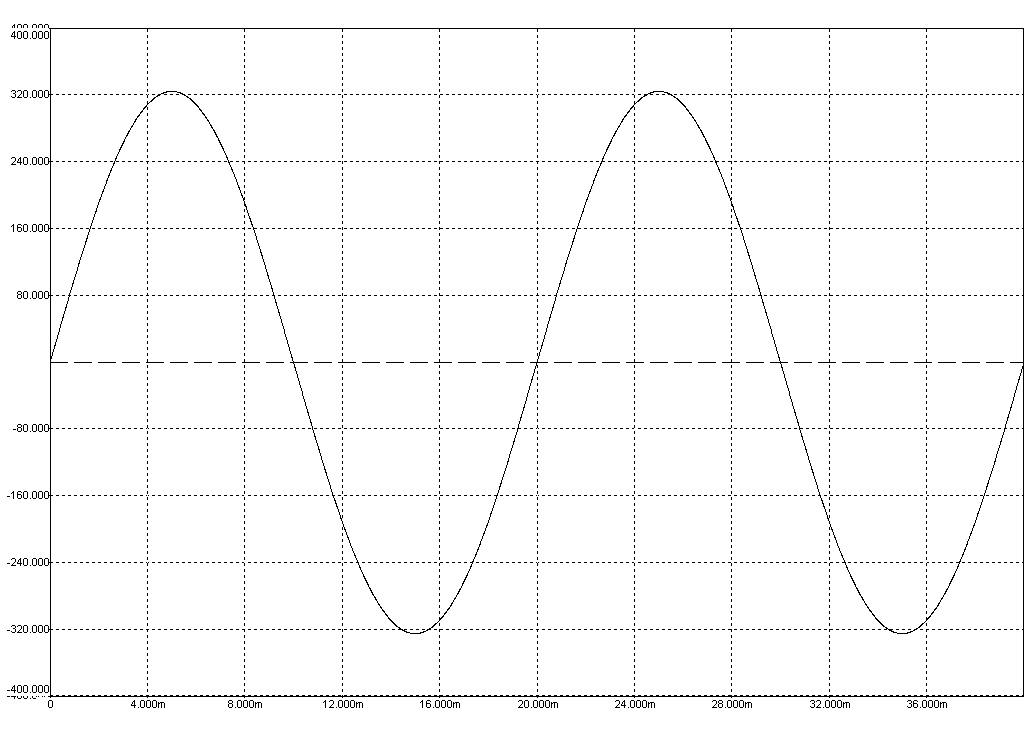• Trace:Output at (SINUSOIDAL1)

SCOPE2: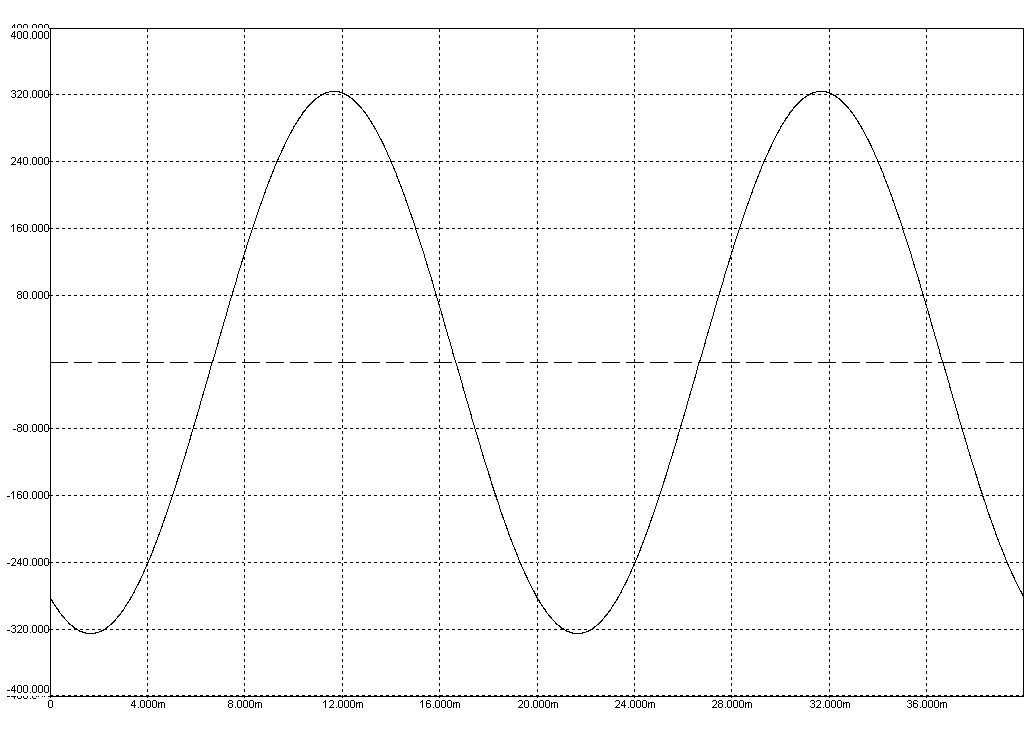• Trace:Output at (SINUSOIDAL2)

SCOPE3:• Trace:Output at (SQUAREWAVE)

SCOPE4: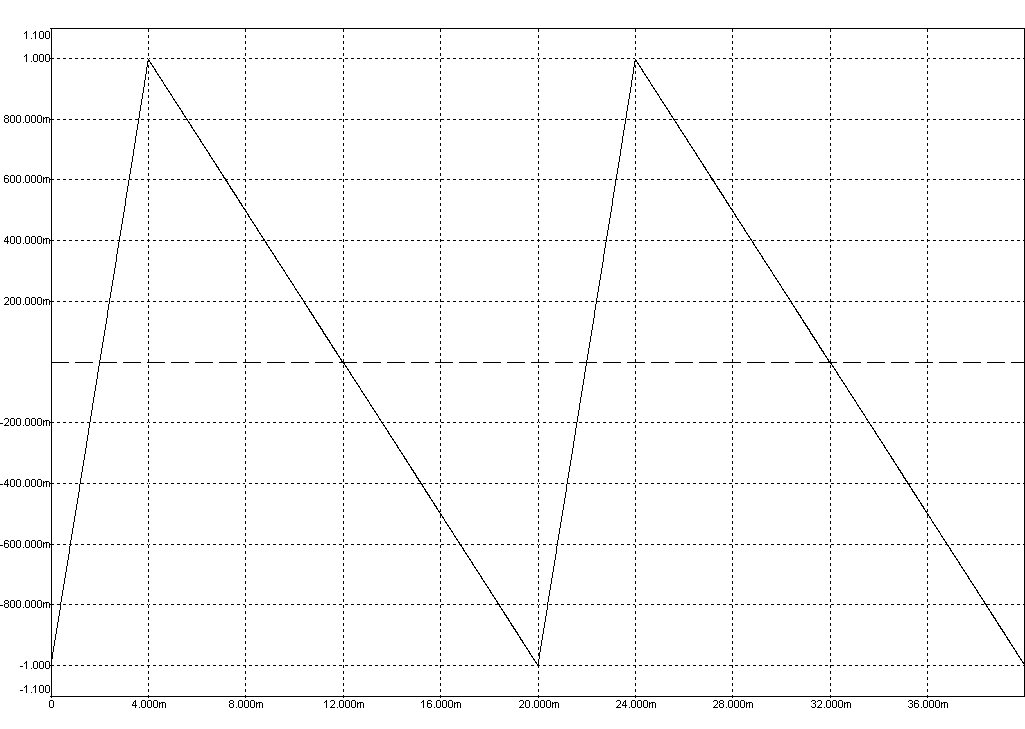• Trace:Output at (TRIANGLE)

SCOPE5: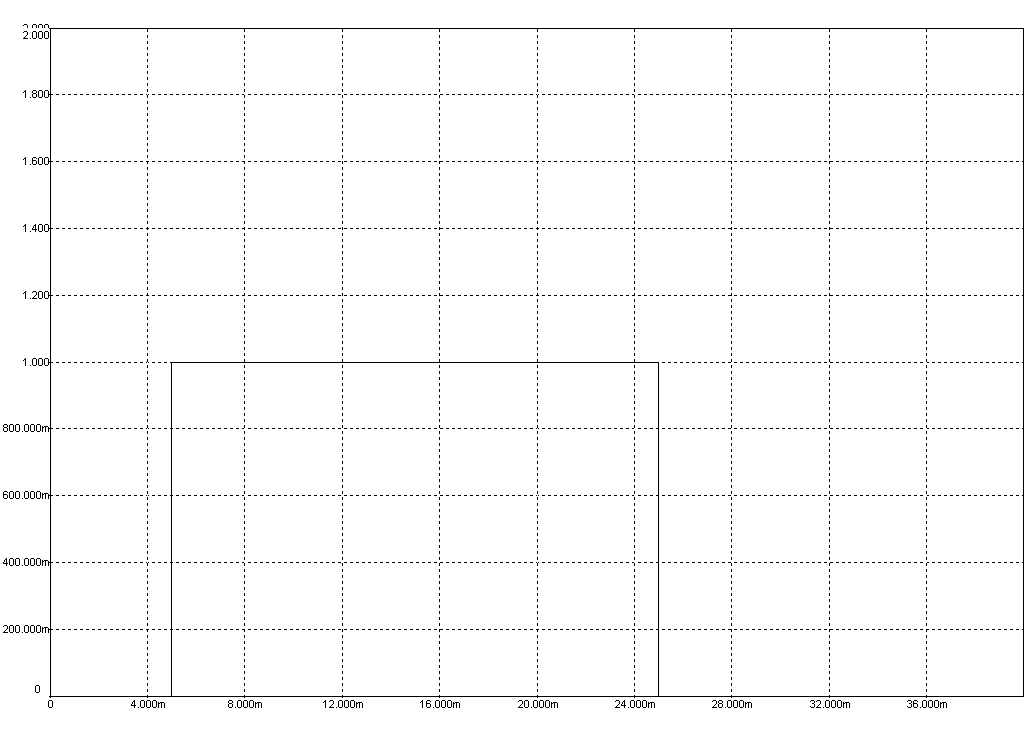• Trace:Output at (PULSE)

SCOPE6:• Trace:Output at (STEP)

SCOPE7:• Trace:Output at (SAW)

SCOPE8:• Trace:Output at (CENTER)

SCOPE9:• Trace:Output at (TRAPEZOIDAL)